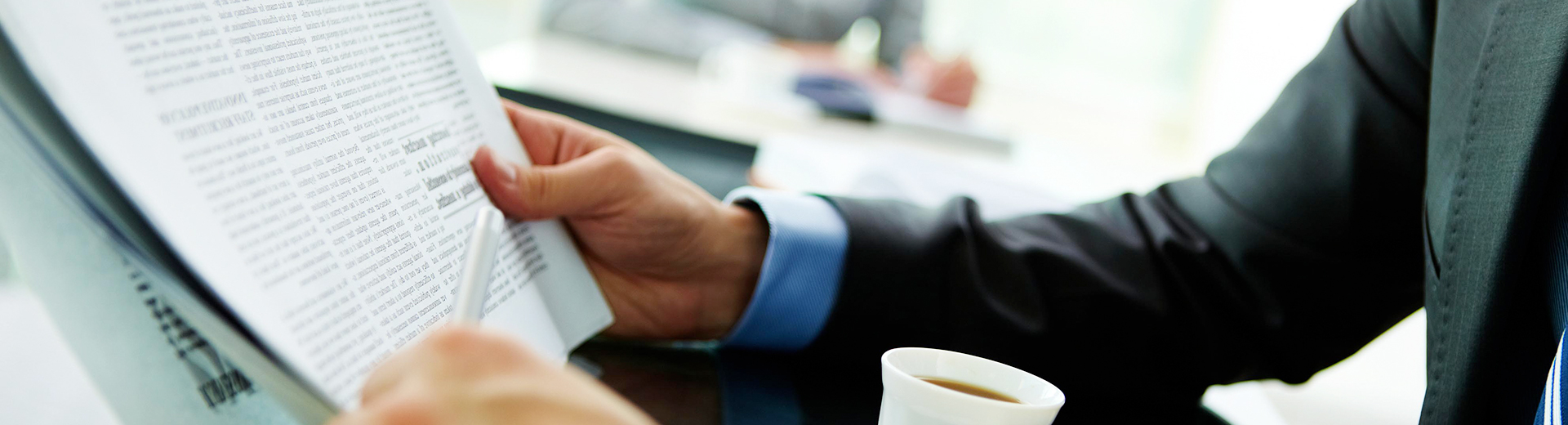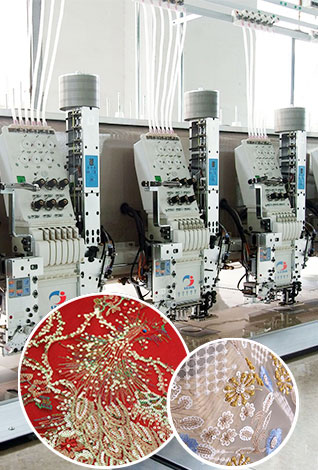# The components of the stepper motor of the computerized embroidery machine

Update:23-12-2020
Summary: The stepping motor of the computerized embroidery machine is composed of three elements: step angle (involving the numb...
The stepping motor of the computerized embroidery machine is composed of three elements: step angle (involving the number of phases), static torque, and current. Once the three elements are determined, the model of the stepper motor of the computerized embroidery machine is determined.
1. The choice of step angle. The step angle of the motor depends on the requirements of load accuracy. Convert the minimum resolution (equivalent) of the load to the motor shaft, and how many angles each equivalent motor should travel (including deceleration). The step angle of the motor should be equal to or less than this angle. At present, the step angle of the stepper motor of the computerized embroidery machine on the market generally has 0.36 degrees/0.72 degrees (five-phase motor), 0.9 degrees/1.8 degrees (two and four-phase motors), 1.5 degrees/3 degrees (three-phase motors), etc.2. The choice of static torque. It is difficult to determine the dynamic torque of the stepping motor of the computerized embroidery machine at once. We often determine the static torque of the motor first. The selection of static torque is based on the load of the motor, and the load can be divided into inertial load and friction load. There is no single inertial load and single friction load. Both loads must be considered when starting directly (generally from low speed). Inertial load is mainly considered when accelerating and starting, and friction load is only considered when running at constant speed. In general, the static torque should be within 2-3 times of the friction load. Once the static torque is selected, the frame and length of the motor can be determined (geometric size)
3. The choice of current. Motors with the same static torque have very different operating characteristics due to different current parameters. You can judge the current of the motor based on the torque-frequency characteristic curve (refer to the drive power and drive voltage). In summary, the following steps should be followed when selecting a motor.
Conversion of torque and power. Stepper motors of computerized embroidery machines are generally used for speed regulation in a relatively large range, and their power is variable. Generally speaking, only torque is used to measure. Torque and power are converted as follows: p=ω·m, ω=2π·n/60, p =2πnm/60 where p is the power unit in watts, ω is the angular velocity per second in radians, n is the speed per minute, m is the torque unit in Newton·m p=2πfm/400 (half-step work) where f is every Number of pulses per second (referred to as pps).

Copyright Zhejiang Lejia Electrical Machinery Co., Ltd. All Rights Reserved.